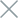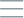• Home
• Tags
• Series
•# Graph Search Algorithm in C++ - Explanation, Example and Code

The Graph Search algorithm is a fundamental technique in the field of graph processing and information retrieval. This algorithm enables us to find paths or components in a graph based on specific rules or search algorithms.

## How It Works

1. Start from a specific vertex (node) in the graph.
2. Perform the search process based on specific rules, such as Depth-First Search (DFS) or Breadth-First Search (BFS).
3. Traverse the vertices and edges of the graph to search for the target or objects to find.
4. Record the path or search results.

## Example

Consider the following graph:

``````A -- B -- C -- E
|         |
D --------
``````

We want to find a path from vertex A to vertex E in this graph using the Depth-First Search (DFS) algorithm.

1. Start at vertex A.
2. Move to vertex B.
3. Continue to vertex C.
4. There are no neighbors in C, backtrack to vertex B.
5. Move to vertex D.
6. Continue to vertex A (as D is connected to A).
7. Move to vertex B.
8. Move to vertex C.
9. Move to vertex E.

The path from A to E is A -> B -> C -> E.

## Example Code in C++

``````#include <iostream>
#include <vector>
#include <stack>
#include <unordered_map>

class Graph {
public:
std::vector<char> depthFirstSearch(char start, char end);

private:
};

void Graph::addEdge(char from, char to) {
}

std::vector<char> Graph::depthFirstSearch(char start, char end) {
std::vector<char> path;
std::unordered_map<char, char> parent;
std::stack<char> stack;

stack.push(start);
parent[start] = '\0';

while (!stack.empty()) {
char current = stack.top();
stack.pop();

if (current == end) {
// Build the path from end to start using the parent map
char node = end;
while (node != '\0') {
path.insert(path.begin(), node);
node = parent[node];
}
break;
}

for (char neighbor : adjList[current]) {
if (parent.find(neighbor) == parent.end()) {
parent[neighbor] = current;
stack.push(neighbor);
}
}
}

return path;
}

int main() {
Graph graph;

char start = 'A';
char end = 'E';

std::vector<char> path = graph.depthFirstSearch(start, end);

std::cout << "Path from " << start << " to " << end << ": ";
for (char node : path) {
std::cout << node << " ";
}
std::cout << std::endl;

return 0;
}
``````

In this example, we use the DFS algorithm to find a path from vertex A to vertex E in the graph. The result will be a sequence of vertices forming the path from A to E.